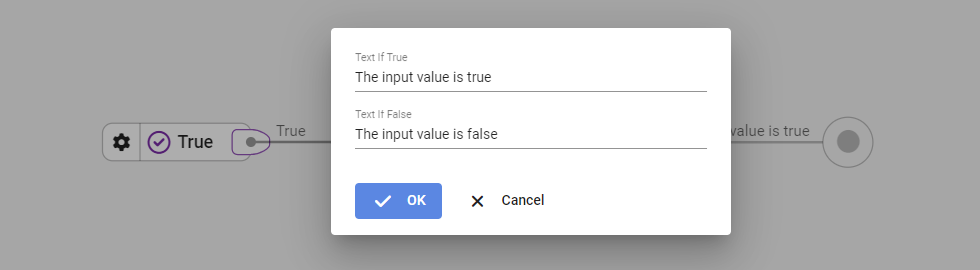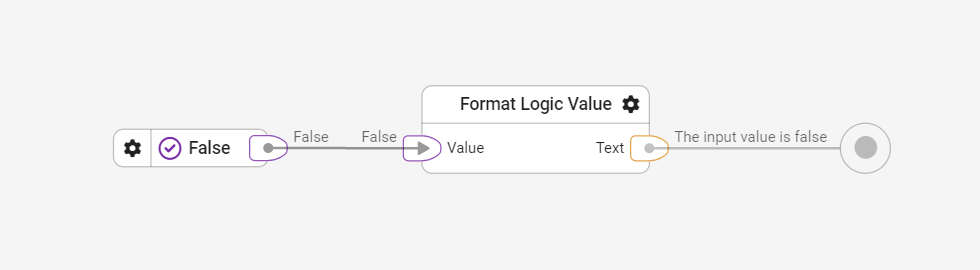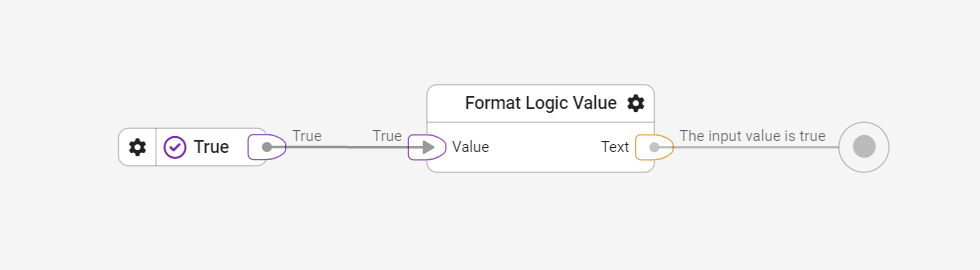Logic

# Format a logic value using the Format Logic Value block

Format a logic value

The Format Logic Value block is used to format a logic value to a text.

Example A:Clicking on the gear icon opens a dialog where the return values for true and for false can be configured as text.

Example B:The result of input value 'False' is 'The input value is false'.

Example C:The result of input value 'True' is 'The input value is true'.

Result:

Returns the configured text value depending on the input type true or false.

Tips and best practices:

-

Library:

Logic